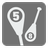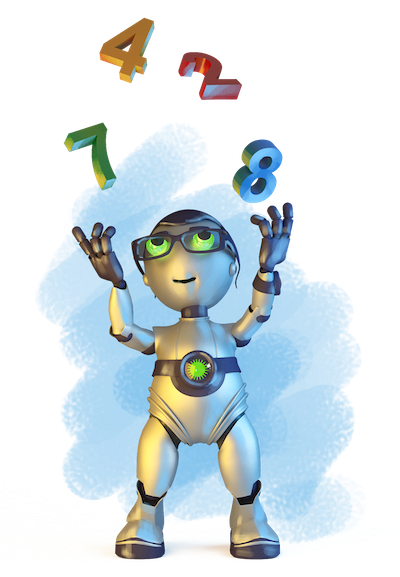Digits Multiplication
Elementary+
English DE EL FR HU IT JA RU UK ZH-HANS
We have prepared a set of Editor's Choice Solutions. You will see them first after you solve the mission. In order to see all other solutions you should change the filter.You are given a positive integer. Your function should calculate the product of the digits excluding any zeroes.

For example: The number given is 123405. The result will be 1*2*3*4*5=120 (don't forget to exclude zeroes).

Input: A positive integer.

Output: The product of the digits as an integer.

Example:

```checkio(123405) == 120
checkio(999) == 729
checkio(1000) == 1
checkio(1111) == 1
```

How it is used: This task can teach you how to solve a problem with simple data type conversion.

Precondition: 0 < number < 106